# design of Spur gear

Mar. 26, 2023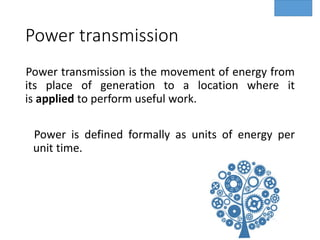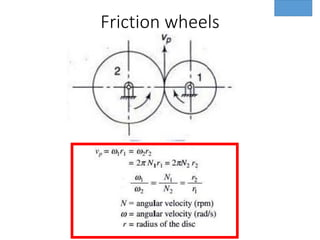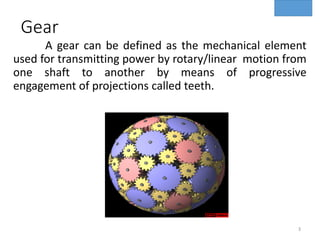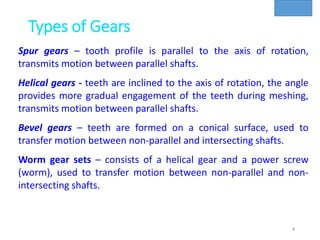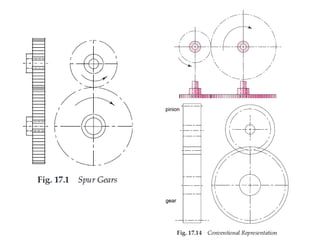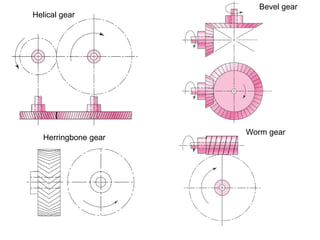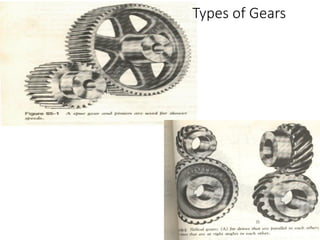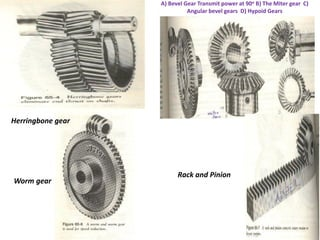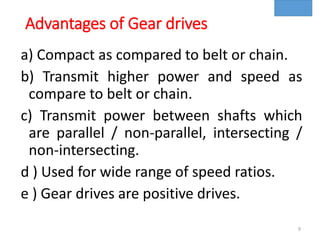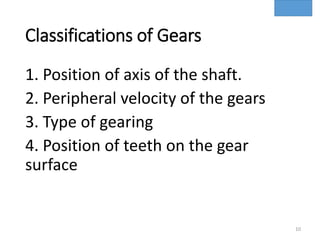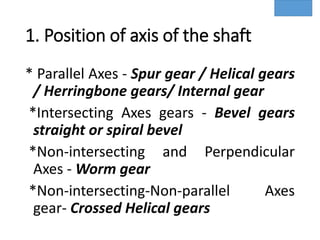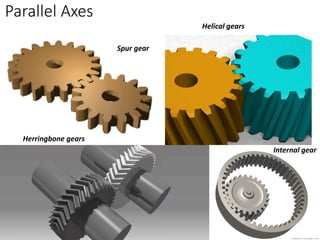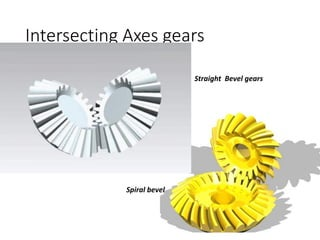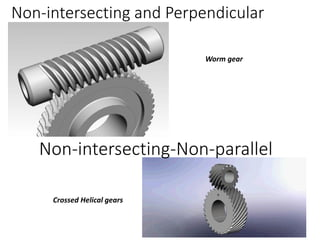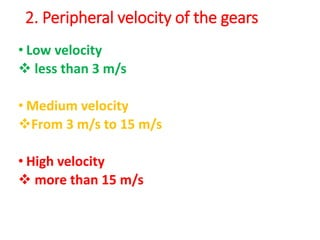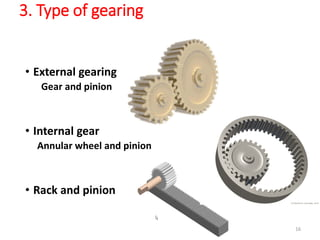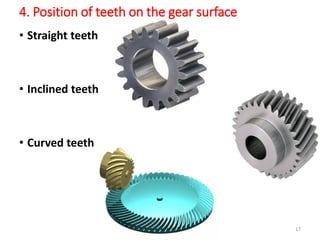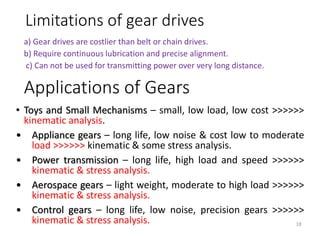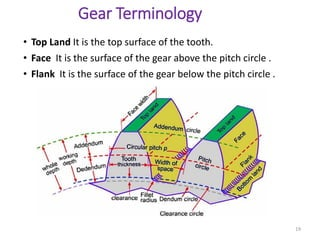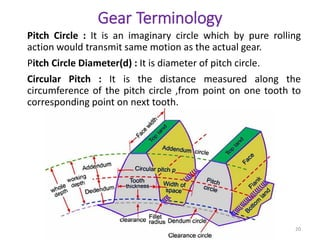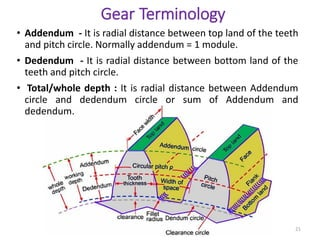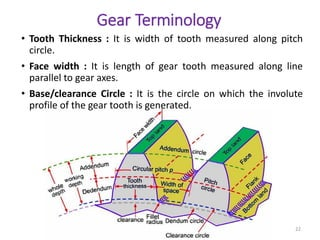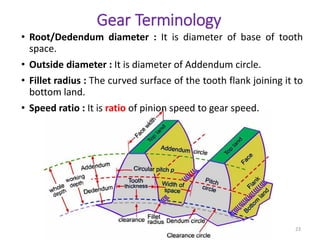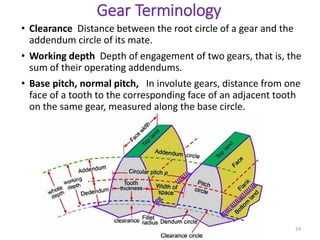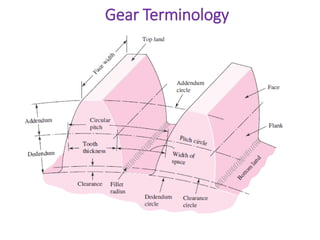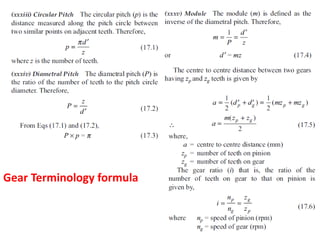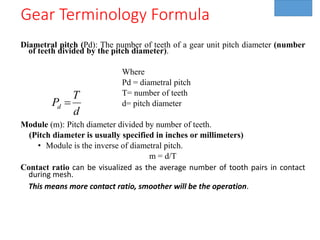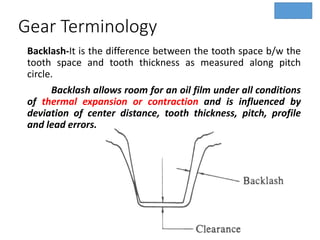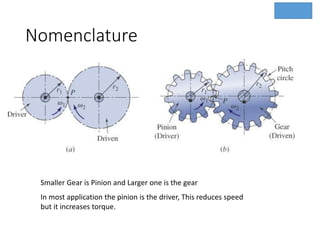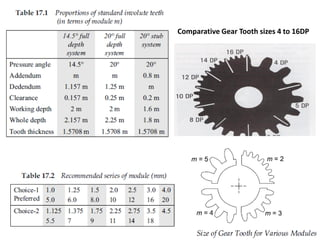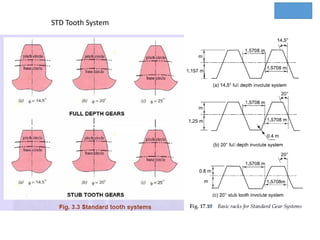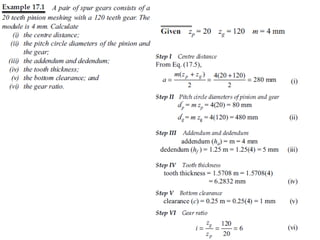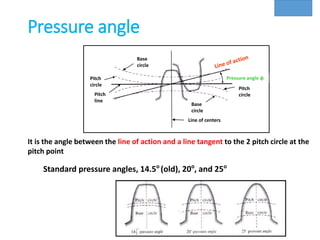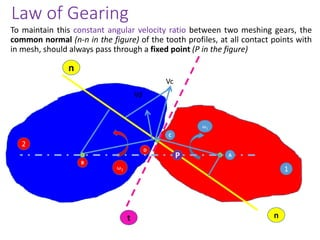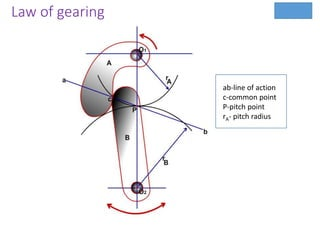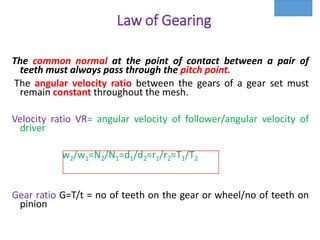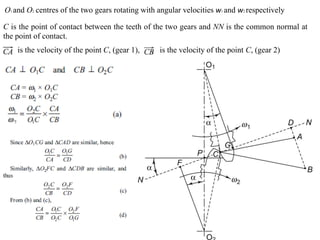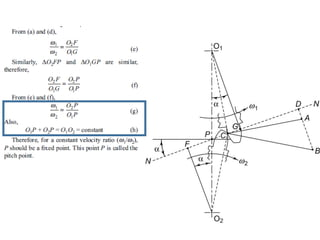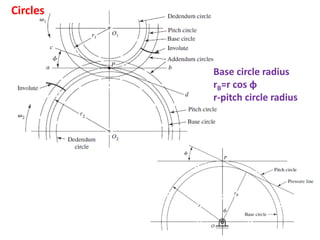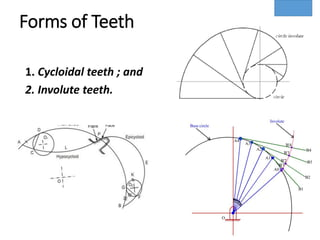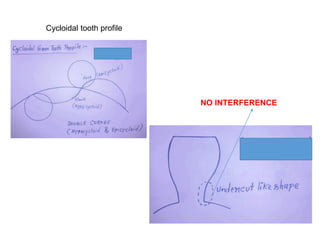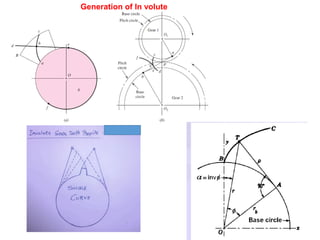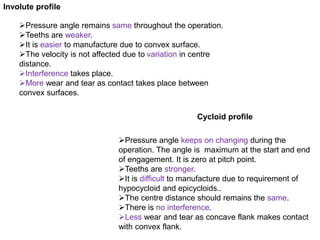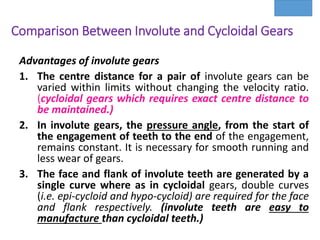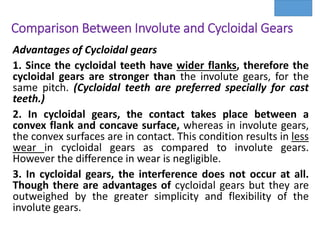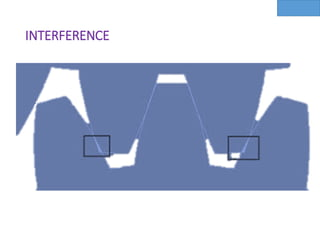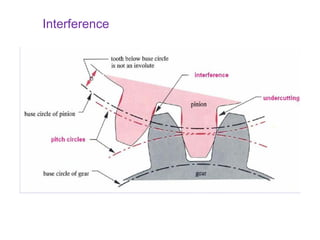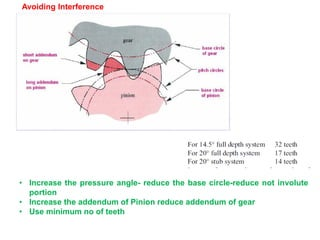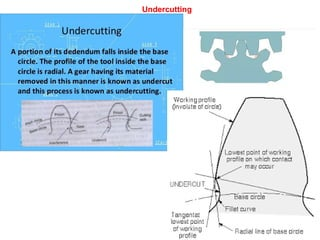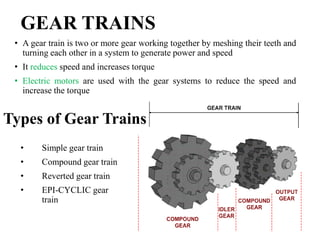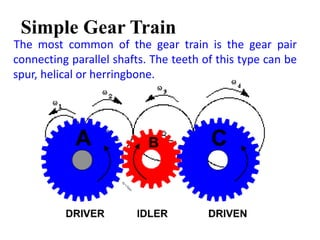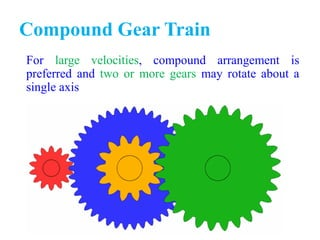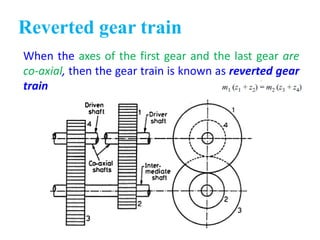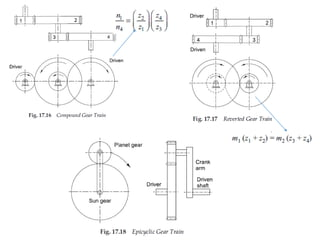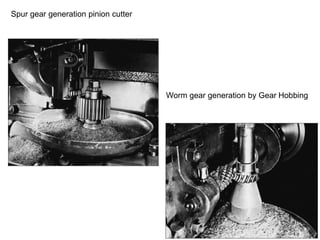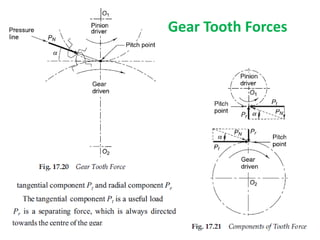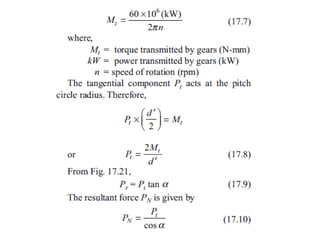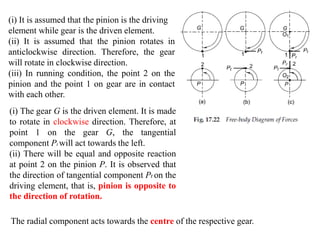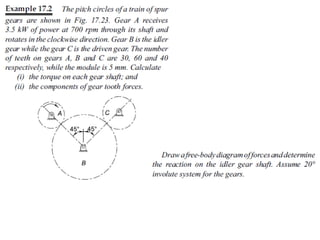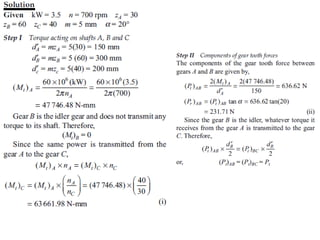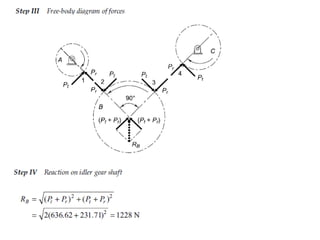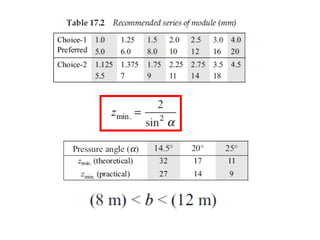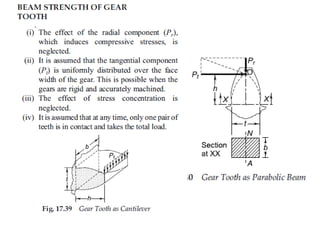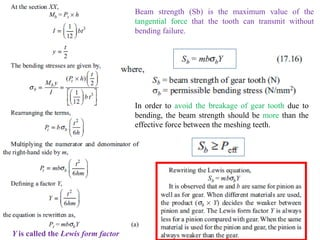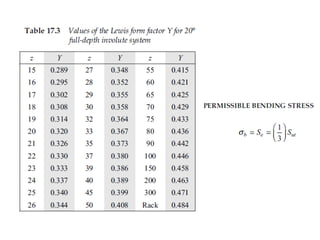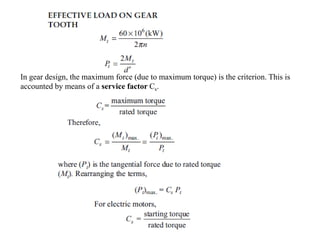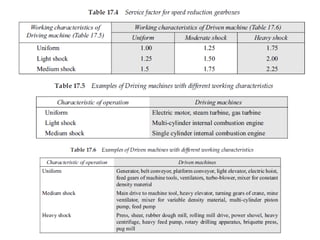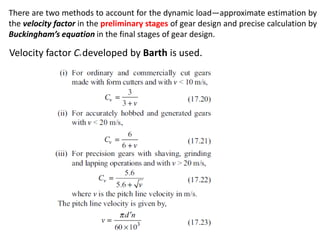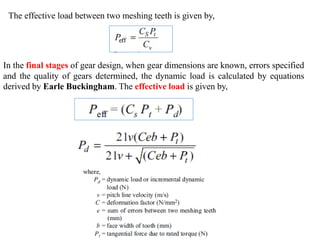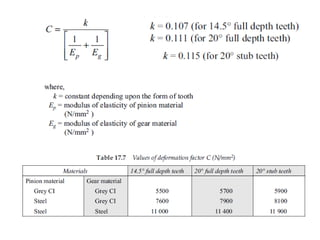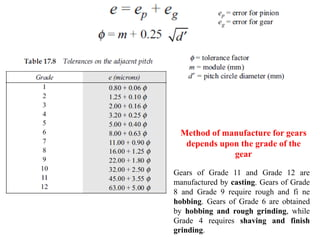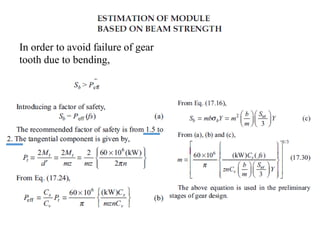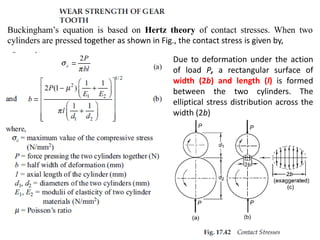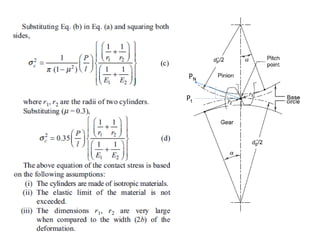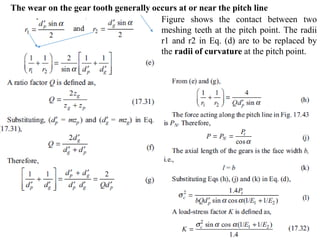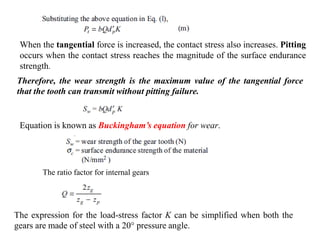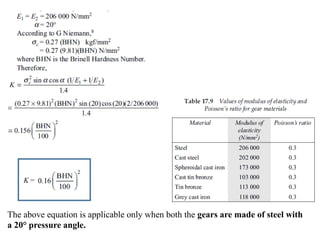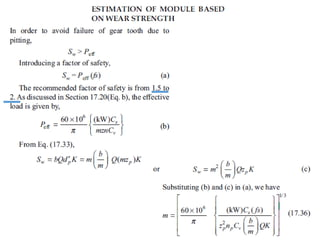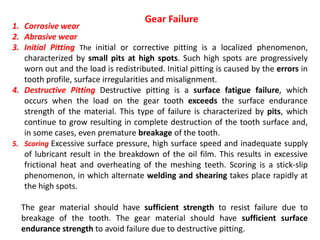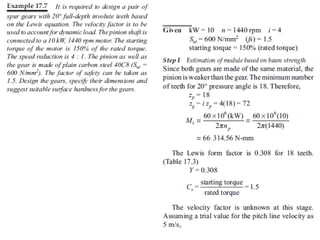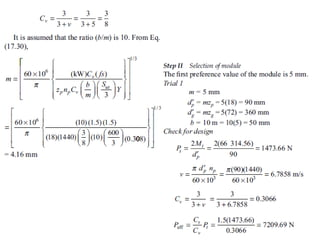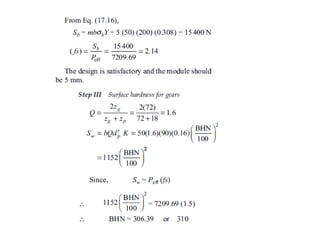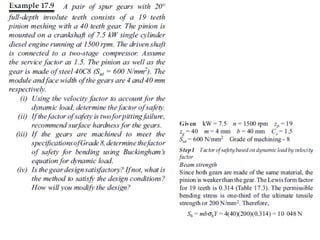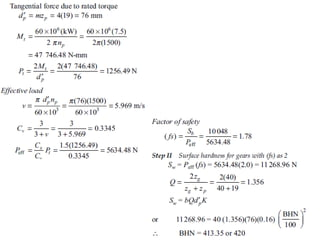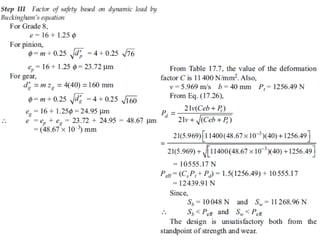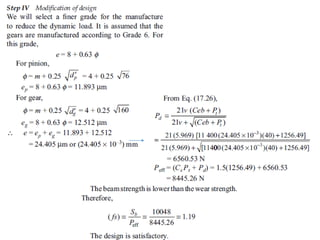1 of 86

### design of Spur gear

1. Power transmission Power transmission is the movement of energy from its place of generation to a location where it is applied to perform useful work. Power is defined formally as units of energy per unit time.
2. Friction wheels
3. A gear can be defined as the mechanical element used for transmitting power by rotary/linear motion from one shaft to another by means of progressive engagement of projections called teeth. 3 Gear
4. Types of Gears 4 Spur gears – tooth profile is parallel to the axis of rotation, transmits motion between parallel shafts. Helical gears - teeth are inclined to the axis of rotation, the angle provides more gradual engagement of the teeth during meshing, transmits motion between parallel shafts. Bevel gears – teeth are formed on a conical surface, used to transfer motion between non-parallel and intersecting shafts. Worm gear sets – consists of a helical gear and a power screw (worm), used to transfer motion between non-parallel and non- intersecting shafts.
5. Helical gear Bevel gear Worm gear Herringbone gear
6. Types of Gears
7. A) Bevel Gear Transmit power at 90o B) The Miter gear C) Angular bevel gears D) Hypoid Gears Worm gear Rack and Pinion Herringbone gear
8. Advantages of Gear drives a) Compact as compared to belt or chain. b) Transmit higher power and speed as compare to belt or chain. c) Transmit power between shafts which are parallel / non-parallel, intersecting / non-intersecting. d ) Used for wide range of speed ratios. e ) Gear drives are positive drives. 9
9. Classifications of Gears 1. Position of axis of the shaft. 2. Peripheral velocity of the gears 3. Type of gearing 4. Position of teeth on the gear surface 10
10. 1. Position of axis of the shaft * Parallel Axes - Spur gear / Helical gears / Herringbone gears/ Internal gear *Intersecting Axes gears - Bevel gears straight or spiral bevel *Non-intersecting and Perpendicular Axes - Worm gear *Non-intersecting-Non-parallel Axes gear- Crossed Helical gears
11. Parallel Axes Spur gear Helical gears Herringbone gears Internal gear
12. Intersecting Axes gears Straight Bevel gears Spiral bevel
13. Non-intersecting and Perpendicular Worm gear Non-intersecting-Non-parallel Crossed Helical gears
14. 2. Peripheral velocity of the gears • Low velocity ❖ less than 3 m/s • Medium velocity ❖From 3 m/s to 15 m/s • High velocity ❖ more than 15 m/s
15. 3. Type of gearing • External gearing Gear and pinion • Internal gear Annular wheel and pinion • Rack and pinion 16
16. 4. Position of teeth on the gear surface • Straight teeth • Inclined teeth • Curved teeth 17
17. Limitations of gear drives a) Gear drives are costlier than belt or chain drives. b) Require continuous lubrication and precise alignment. c) Can not be used for transmitting power over very long distance. 18 • Toys and Small Mechanisms – small, low load, low cost >>>>>> kinematic analysis. • Appliance gears – long life, low noise & cost low to moderate load >>>>>> kinematic & some stress analysis. • Power transmission – long life, high load and speed >>>>>> kinematic & stress analysis. • Aerospace gears – light weight, moderate to high load >>>>>> kinematic & stress analysis. • Control gears – long life, low noise, precision gears >>>>>> kinematic & stress analysis. Applications of Gears
18. Gear Terminology • Top Land It is the top surface of the tooth. • Face It is the surface of the gear above the pitch circle . • Flank It is the surface of the gear below the pitch circle . 19
19. Gear Terminology Pitch Circle : It is an imaginary circle which by pure rolling action would transmit same motion as the actual gear. Pitch Circle Diameter(d) : It is diameter of pitch circle. Circular Pitch : It is the distance measured along the circumference of the pitch circle ,from point on one tooth to corresponding point on next tooth. 20
20. Gear Terminology • Addendum - It is radial distance between top land of the teeth and pitch circle. Normally addendum = 1 module. • Dedendum - It is radial distance between bottom land of the teeth and pitch circle. • Total/whole depth : It is radial distance between Addendum circle and dedendum circle or sum of Addendum and dedendum. 21
21. Gear Terminology • Tooth Thickness : It is width of tooth measured along pitch circle. • Face width : It is length of gear tooth measured along line parallel to gear axes. • Base/clearance Circle : It is the circle on which the involute profile of the gear tooth is generated. 22
22. Gear Terminology • Root/Dedendum diameter : It is diameter of base of tooth space. • Outside diameter : It is diameter of Addendum circle. • Fillet radius : The curved surface of the tooth flank joining it to bottom land. • Speed ratio : It is ratio of pinion speed to gear speed. 23
23. Gear Terminology • Clearance Distance between the root circle of a gear and the addendum circle of its mate. • Working depth Depth of engagement of two gears, that is, the sum of their operating addendums. • Base pitch, normal pitch, In involute gears, distance from one face of a tooth to the corresponding face of an adjacent tooth on the same gear, measured along the base circle. 24
24. Gear Terminology
25. Gear Terminology formula
26. Diametral pitch (Pd): The number of teeth of a gear unit pitch diameter (number of teeth divided by the pitch diameter). Where Pd = diametral pitch T= number of teeth d= pitch diameter Module (m): Pitch diameter divided by number of teeth. (Pitch diameter is usually specified in inches or millimeters) • Module is the inverse of diametral pitch. m = d/T Contact ratio can be visualized as the average number of tooth pairs in contact during mesh. This means more contact ratio, smoother will be the operation. d T Pd = Gear Terminology Formula
27. Backlash-It is the difference between the tooth space b/w the tooth space and tooth thickness as measured along pitch circle. Backlash allows room for an oil film under all conditions of thermal expansion or contraction and is influenced by deviation of center distance, tooth thickness, pitch, profile and lead errors. Gear Terminology
28. Nomenclature Smaller Gear is Pinion and Larger one is the gear In most application the pinion is the driver, This reduces speed but it increases torque.
29. Comparative Gear Tooth sizes 4 to 16DP
30. STD Tooth System
31. Pressure angle Pitch line Line of centers Base circle Base circle Pitch circle Pitch circle Pressure angle φ Standard pressure angles, 14.5o (old), 20o , and 25o It is the angle between the line of action and a line tangent to the 2 pitch circle at the pitch point
32. Law of Gearing 2 1 D C n n ω1 ω2 A B t P Vc Vd To maintain this constant angular velocity ratio between two meshing gears, the common normal (n-n in the figure) of the tooth profiles, at all contact points with in mesh, should always pass through a fixed point (P in the figure)
33. Law of gearing ab-line of action c-common point P-pitch point rA- pitch radius
34. Law of Gearing The common normal at the point of contact between a pair of teeth must always pass through the pitch point. The angular velocity ratio between the gears of a gear set must remain constant throughout the mesh. Velocity ratio VR= angular velocity of follower/angular velocity of driver w2/w1=N2/N1=d1/d2=r1/r2=T1/T2 Gear ratio G=T/t = no of teeth on the gear or wheel/no of teeth on pinion
35. O1 and O2 centres of the two gears rotating with angular velocities w1 and w2 respectively C is the point of contact between the teeth of the two gears and NN is the common normal at the point of contact. is the velocity of the point C, (gear 1), is the velocity of the point C, (gear 2)
37. Forms of Teeth 1. Cycloidal teeth ; and 2. Involute teeth.
38. Cycloidal tooth profile NO INTERFERENCE
39. Generation of In volute
40. ➢Pressure angle remains same throughout the operation. ➢Teeths are weaker. ➢It is easier to manufacture due to convex surface. ➢The velocity is not affected due to variation in centre distance. ➢Interference takes place. ➢More wear and tear as contact takes place between convex surfaces. ➢Pressure angle keeps on changing during the operation. The angle is maximum at the start and end of engagement. It is zero at pitch point. ➢Teeths are stronger. ➢It is difficult to manufacture due to requirement of hypocycloid and epicycloids.. ➢The centre distance should remains the same. ➢There is no interference. ➢Less wear and tear as concave flank makes contact with convex flank. Involute profile Cycloid profile
41. Comparison Between Involute and Cycloidal Gears Advantages of involute gears 1. The centre distance for a pair of involute gears can be varied within limits without changing the velocity ratio. (cycloidal gears which requires exact centre distance to be maintained.) 2. In involute gears, the pressure angle, from the start of the engagement of teeth to the end of the engagement, remains constant. It is necessary for smooth running and less wear of gears. 3. The face and flank of involute teeth are generated by a single curve where as in cycloidal gears, double curves (i.e. epi-cycloid and hypo-cycloid) are required for the face and flank respectively. (involute teeth are easy to manufacture than cycloidal teeth.)
42. Comparison Between Involute and Cycloidal Gears Advantages of Cycloidal gears 1. Since the cycloidal teeth have wider flanks, therefore the cycloidal gears are stronger than the involute gears, for the same pitch. (Cycloidal teeth are preferred specially for cast teeth.) 2. In cycloidal gears, the contact takes place between a convex flank and concave surface, whereas in involute gears, the convex surfaces are in contact. This condition results in less wear in cycloidal gears as compared to involute gears. However the difference in wear is negligible. 3. In cycloidal gears, the interference does not occur at all. Though there are advantages of cycloidal gears but they are outweighed by the greater simplicity and flexibility of the involute gears.
43. INTERFERENCE
44. Interference
45. Avoiding Interference • Increase the pressure angle- reduce the base circle-reduce not involute portion • Increase the addendum of Pinion reduce addendum of gear • Use minimum no of teeth
46. Undercutting
47. GEAR TRAINS • A gear train is two or more gear working together by meshing their teeth and turning each other in a system to generate power and speed • It reduces speed and increases torque • Electric motors are used with the gear systems to reduce the speed and increase the torque • Simple gear train • Compound gear train • Reverted gear train • EPI-CYCLIC gear train Types of Gear Trains
48. Simple Gear Train The most common of the gear train is the gear pair connecting parallel shafts. The teeth of this type can be spur, helical or herringbone.
49. Compound Gear Train For large velocities, compound arrangement is preferred and two or more gears may rotate about a single axis
50. Reverted gear train When the axes of the first gear and the last gear are co-axial, then the gear train is known as reverted gear train
51. Spur gear generation pinion cutter Worm gear generation by Gear Hobbing
52. Gear Tooth Forces
53. (i) It is assumed that the pinion is the driving element while gear is the driven element. (ii) It is assumed that the pinion rotates in anticlockwise direction. Therefore, the gear will rotate in clockwise direction. (iii) In running condition, the point 2 on the pinion and the point 1 on gear are in contact with each other. (i) The gear G is the driven element. It is made to rotate in clockwise direction. Therefore, at point 1 on the gear G, the tangential component Pt will act towards the left. (ii) There will be equal and opposite reaction at point 2 on the pinion P. It is observed that the direction of tangential component Pt on the driving element, that is, pinion is opposite to the direction of rotation. The radial component acts towards the centre of the respective gear.
54. In order to avoid the breakage of gear tooth due to bending, the beam strength should be more than the effective force between the meshing teeth. Y is called the Lewis form factor Beam strength (Sb) is the maximum value of the tangential force that the tooth can transmit without bending failure.
55. In gear design, the maximum force (due to maximum torque) is the criterion. This is accounted by means of a service factor Cs.
56. There are two methods to account for the dynamic load—approximate estimation by the velocity factor in the preliminary stages of gear design and precise calculation by Buckingham’s equation in the final stages of gear design. Velocity factor Cv developed by Barth is used.
57. In the final stages of gear design, when gear dimensions are known, errors specified and the quality of gears determined, the dynamic load is calculated by equations derived by Earle Buckingham. The effective load is given by, The effective load between two meshing teeth is given by,
58. Gears of Grade 11 and Grade 12 are manufactured by casting. Gears of Grade 8 and Grade 9 require rough and fi ne hobbing. Gears of Grade 6 are obtained by hobbing and rough grinding, while Grade 4 requires shaving and finish grinding. Method of manufacture for gears depends upon the grade of the gear
59. In order to avoid failure of gear tooth due to bending,
60. Buckingham’s equation is based on Hertz theory of contact stresses. When two cylinders are pressed together as shown in Fig., the contact stress is given by, Due to deformation under the action of load P, a rectangular surface of width (2b) and length (l) is formed between the two cylinders. The elliptical stress distribution across the width (2b)
61. PN Pt
62. Figure shows the contact between two meshing teeth at the pitch point. The radii r1 and r2 in Eq. (d) are to be replaced by the radii of curvature at the pitch point. The wear on the gear tooth generally occurs at or near the pitch line
63. Therefore, the wear strength is the maximum value of the tangential force that the tooth can transmit without pitting failure. Equation is known as Buckingham’s equation for wear. When the tangential force is increased, the contact stress also increases. Pitting occurs when the contact stress reaches the magnitude of the surface endurance strength. The ratio factor for internal gears The expression for the load-stress factor K can be simplified when both the gears are made of steel with a 20° pressure angle.
64. The above equation is applicable only when both the gears are made of steel with a 20° pressure angle.
65. The gear material should have sufficient strength to resist failure due to breakage of the tooth. The gear material should have sufficient surface endurance strength to avoid failure due to destructive pitting. 1. Corrosive wear 2. Abrasive wear 3. Initial Pitting The initial or corrective pitting is a localized phenomenon, characterized by small pits at high spots. Such high spots are progressively worn out and the load is redistributed. Initial pitting is caused by the errors in tooth profile, surface irregularities and misalignment. 4. Destructive Pitting Destructive pitting is a surface fatigue failure, which occurs when the load on the gear tooth exceeds the surface endurance strength of the material. This type of failure is characterized by pits, which continue to grow resulting in complete destruction of the tooth surface and, in some cases, even premature breakage of the tooth. 5. Scoring Excessive surface pressure, high surface speed and inadequate supply of lubricant result in the breakdown of the oil film. This results in excessive frictional heat and overheating of the meshing teeth. Scoring is a stick-slip phenomenon, in which alternate welding and shearing takes place rapidly at the high spots. Gear Failure